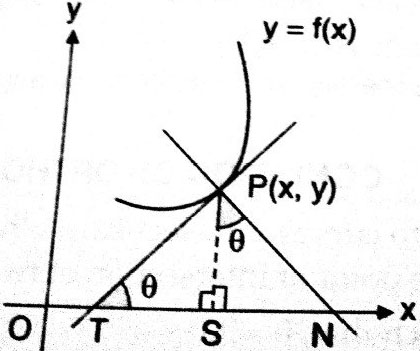# Let a tangent to any curve at a point P on it cuts  at N. If a & b are lenght of subtangent and subnormal then a + b equals ? Option 1) Option 2) Option 3) Option 4)

H Himanshu

As we have learned

Concept of sub Tangent and sub Normal -

When Tangent and Normal cuts the x-axis at a point and perpendicular from point of contact to x-axis.Then this is sub Normal & Tangent.From fig TS  and SN are known as sub tangent and sub normal.

- whereinOption 1)

Option 2)

Option 3)

Option 4)

Exams
Articles
Questions